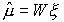April / Monthly / Tutorials / Software / Home
 In a typical state-of-the-art large vocabulary conversational speech recognition (LVCSR) system, a single speech model is developed using data from a large number of speakers to cover the variance across dialect, speaking styles, etc. Since the speech model is the average of all the speakers, the speech recognition results should be the average of all speakers. Such system is called a speaker independent system. The drawback of such a system is that its performance is not optimal for any particular speaker. To make the recognition system perform optimal for a particular speaker, the best paradigm is to construct a system using all the data only collected from this speaker. Such a system is called speaker dependent system. The speaker dependent system usually performs badly for other speakers. At the same time collecting a lot of data from one speaker is a very difficult task. The obvious solution is that we can use the speaker independent system, by collecting a small amount of data from a new speaker. The system can then be adapted to fit specific feature of the new speaker. The new system, thus created, will give better performance for this speaker. The performance of the new system will lie between the speaker independent system and speaker dependent system. The more adaptation data, the more closer to speaker dependent system. The Maximum Likelihood Linear Regression (MLLR) can be used to perform such an adaptation, and it will be released with version r00_n12 of our production system. You can monitor the progress of this release using our asr mailing list. This tutorial provides steps on how to run our production system using MLLR adaptation. The theory behind this implementation can be found in the dissertation: Chris J. Leggetter, Improved Acoustic Modeling for HMMs Using Linear Transformations, PhD thesis, Department of Engineering, University of Cambridge. February 1995, Commonly, it is assumed that the primary difference between speakers is in the average position of phones in the acoustic space. In other words, the mean adaptation gives profound performance. Currently, we have only implemented the mean adaptation and in this tutorial, we mainly discuss the mean adaptation. By using some given adaptation data, MLLR can build a single global transform to adapt all models. We can use following equation to get a new estimate of the adapted mean for a model:where n is the dimensionality of the data, W is the n x (n+1) transformation matrix andis the extended mean vector.is the offset indicator, usually set to 1.0. Estimating the transformation matrix (W) is the core of the MLLR adaptation. As more data becomes available, we can do better by classifying the models into different classes and getting fine-grained transformations for each class. A regress class tree plays a critical role to manage those processes. According to the amount, and type of adaptation data available, the set of transformations can be chosen through the regression class tree. In this tutorial, we will cover the process of MLLR adaptation of a speaker and the command line interfaces for MLLR adaptation. The process of MLLR adaptation for a speaker: Using an existing model to conduct MLLR adaptation involves four basic steps according to users' specification. Those are: regression tree generation, adaptation accumulation, transformation creation, and adaptation of models. Regression Tree Generation: The first step in MLLR adaptation is to create regression decision tree. The regression decision tree is constructed in such a way that the Gaussian components, which are close in acoustic space, are put in the same regression class and can be transformed in a similar way. The input for this step is the statistical acoustic models of the system. The output for this step is a regression decision tree. Adaptation Data Accumulation: Next, the adaptation data is accumulated. This step is the same as the general training process of the system. The input for this step is the model and speech data of a specific speaker, and output is models including the adaptation data. Transformation Creation: Then, the regression decision tree and models which accumulated the adaptation data are used to create transformation matrix for each regression class, which actually is the node of the regression decision tree. The input for this step is the regression tree built in the step i. and models included the adaptation information from step ii. The output for this step is the regression tree which includes the transformation matrix for each node. Adaptation: Finally, each component of a model is adapted by a specific transform matrix which belongs to a particular corresponding regression class. The input for this step is the models and regression decision tree, and the output is the adapted models. The command line for MLLR adaptation: All four steps mentioned above can be processed in one command line. The command line for MLLR adaptation is the same as other cases of using isip_recognize. The only difference is in the parameter file. Users need to specify the options for MLLR adaptation in the parameter file. isip_recognize.exe -parameter_file params/params_1.sof -list lists/identifiers.sof -verbose brief Finally, one more note: MLLR decoding is the same as standard decoding, but you need to pay attention to using models that are adapted (output of the adaptation process) for each specific speaker. In this tutorial, we gave a brief introduction for the process for MLLR adaptation and command line interfaces. The MLLR adaptation system usually gets much better performance than a speaker independent system.